NCERT Solutions for Class 10 Maths Exercise 2.3myCBSEguide App

CBSE, NCERT, JEE Main, NEET-UG, NDA, Exam Papers, Question Bank, NCERT Solutions, Exemplars, Revision Notes, Free Videos, MCQ Tests & more.

NCERT solutions for Class 10 Maths Polynomials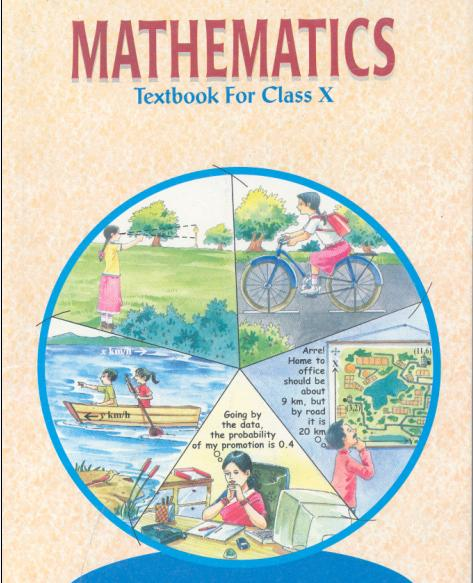NCERT Solutions for Class 10 Maths Polynomials

1. Divide the polynomial p(x) by the polynomial g(x) and find the quotient and remainder in each of the following.

(i)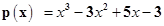,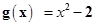(ii)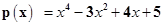,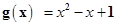(iii)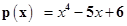,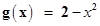Ans. (i)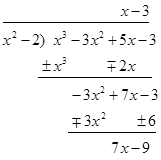Therefore, quotient = x – 3 and Remainder = 7x – 9

(ii)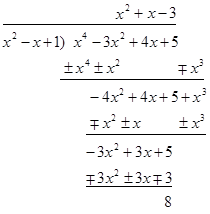Therefore, quotient =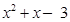and, Remainder = 8

(iii)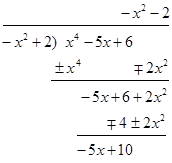Therefore, quotient =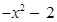and, Remainder = −5x + 10

NCERT Solutions for Class 10 Maths Exercise 2.3

2. Check whether the first polynomial is a factor of the second polynomial by dividing the second polynomial by the first polynomial.

(i)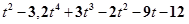(ii)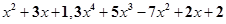(iii)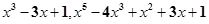Ans. (i)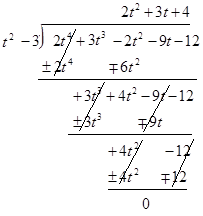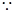Remainder = 0

Hence first polynomial is a factor of second polynomial.

(ii)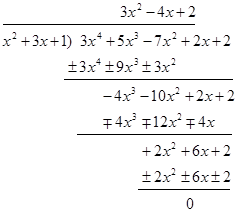Remainder = 0

Hence first polynomial is a factor of second polynomial.

(iii)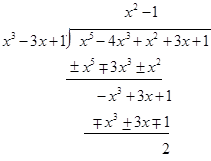Remainder ≠0

Hence first polynomial is not factor of second polynomial.

NCERT Solutions for Class 10 Maths Exercise 2.3

3. Obtain all other zeroes of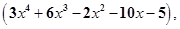if two of its zeroes are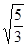and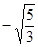.

Ans. Two zeroes of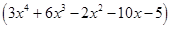areandwhich means that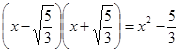is a factor of.

Applying Division Algorithm to find more factors we get: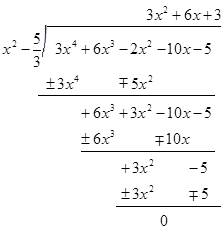We have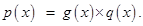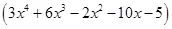= (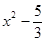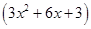)

= ()3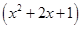= 3()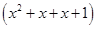= 3()(x+1)(x+1)

Therefore, other two zeroes ofare −1 and −1.

NCERT Solutions for Class 10 Maths Exercise 2.3

4. On dividing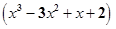by a polynomial g(x), the quotient and remainder were (x-2) and (-2x+4) respectively. Find g(x).

Ans. Let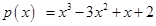, q(x) = (x – 2) and r(x) = (–2x+4)

According to Polynomial Division Algorithm, we have

p(x) = g(x).q(x) + r(x)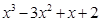= g(x).(x−2)−2x+4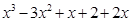−4 = g(x).(x−2)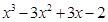= g(x).(x−2)

g(x) =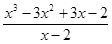So, Dividing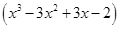by (x−2), we get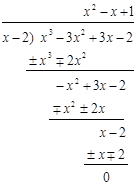Therefore, we have g(x) =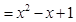NCERT Solutions for Class 10 Maths Exercise 2.3

5. Give examples of polynomials p(x), g(x), q(x) and r(x), which satisfy the division algorithm and

(i) deg p(x) = deg q(x)

(ii) deg q(x) = deg r(x)

(iii) deg r(x) = 0

Ans. (i) Let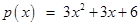, g(x) = 3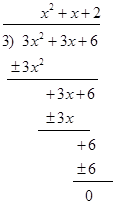So, we can see in this example that deg p(x) = deg q(x) = 2

(ii) Let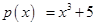and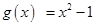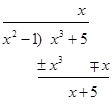We can see in this example that deg q(x) = deg r(x) = 1

(iii) Let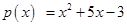, g(x) = x+3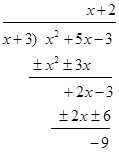We can see in this example that deg r(x) = 0

NCERT Solutions for Class 10 Maths Exercise 2.3

NCERT Solutions Class 10 Maths PDF (Download) Free from myCBSEguide app and myCBSEguide website. Ncert solution class 10 Maths includes text book solutions from Mathematics Book. NCERT Solutions for CBSE Class 10 Maths have total 15 chapters. 10 Maths NCERT Solutions in PDF for free Download on our website. Ncert Maths class 10 solutions PDF and Maths ncert class 10 PDF solutions with latest modifications and as per the latest CBSE syllabus are only available in myCBSEguide.

CBSE app for Class 10

To download NCERT Solutions for Class 10 Maths, Computer Science, Home Science,Hindi ,English, Social Science do check myCBSEguide app or website. myCBSEguide provides sample papers with solution, test papers for chapter-wise practice, NCERT solutions, NCERT Exemplar solutions, quick revision notes for ready reference, CBSE guess papers and CBSE important question papers. Sample Paper all are made available through the best app for CBSE students and myCBSEguide website.16 thoughts on “NCERT Solutions for Class 10 Maths Exercise 2.3”

1. Good solution

2. good solution but first q 2

3. he is best study

4. THANKSQ

thanks a lot..????

6. It is really helpful …….
Thanks a lot …..
?

7. It is really helpful …….
Thanks a lot …..
?

8. It is really helpful …….
Thanks a lot …..
?

9. Amazing solution. …….
Tq ….

10. very nyc and easy to understand…..thank u so much

11. Very helpful……nd easy to understand

12. Good enough…….loved it?

13. Its very useful… Thanks…

14. Thanks a lot . It really helped me to score better marks in our exam.

15. Nice it is so help full

16. It is very helpful for me.
Thank you so much .